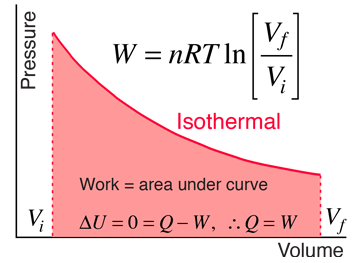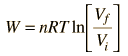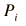# Isothermal Process

 For a constant temperature process involving an ideal gas, pressure can be expressed in terms of the volume:The result of an isothermal heat engine process leading to expansion from Vi to Vf gives the work expression below.Index

Heat engine concepts

 HyperPhysics***** Thermodynamics R Nave
Go Back

# Isothermal ProcessIndex

Heat engine concepts

 HyperPhysics***** Thermodynamics R Nave
Go Back

# Isothermal ProcessFor an ideal gas consisting of n = moles of gas, an isothermal process which involves expansion from

 Vi = m3
 to Vf = m3
 at temperature T = K

The work done in expanding the gas is= J = x10^ J

Applying the ideal gas law in the form shows that for this process= kPa = x10^ Pa= kPa = x10^ Pa
The temperature for the work calculation must be in Kelvins, so it must be converted from the other temperature scales.
 T = K = °C = °F
 Discussion of isothermal process
Index

Heat engine concepts

 HyperPhysics***** Thermodynamics R Nave
Go Back